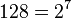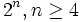# Groups of order 128

## Contents

See pages on algebraic structures of order 128| See pages on groups of a particular order

## Statistics at a glance

To understand these in a broader context, see
groups of order 2^n|groups of prime-seventh order

Note that since prime power order implies nilpotent, and$128 = 2^7$ is a prime power, all groups of order 128 are nilpotent.

Quantity Value Explanation
Number of groups up to isomorphism 2328
Number of abelian groups up to isomorphism 15 Equal to the number of unordered integer partitions of$7$, see classification of finite abelian groups
Number of groups of class exactly two up to isomorphism 947
Number of groups of class exactly three up to isomorphism 1137
Number of groups of class exactly four up to isomorphism 197
Number of groups of class exactly five up to isomorphism 29
Number of maximal class groups, i.e., groups of class exactly six up to isomorphism 3 classification of finite 2-groups of maximal class. For order$2^n, n \ge 4$, there are exactly three maximal class groups: dihedral, semidihedral, and generalized quaternion. For order 128, the groups are: dihedral group:D128, semidihedral group:SD128, and generalized quaternion group:Q128.

## Arithmetic functions

### Summary information

Here, the rows are arithmetic functions that take values between$0$ and$7$, and the columns give the possible values of these functions. The entry in each cell is the number of isomorphism classes of groups for which the row arithmetic function takes the column value. Note that all the row value sums must equal$2328$, which is the total number of groups of order$128$.

Arithmetic function Value 0 Value 1 Value 2 Value 3 Value 4 Value 5 Value 6 Value 7
prime-base logarithm of exponent 0 1 823 1269 202 27 5 1
Frattini length 0 1 816 1276 202 27 5 1
nilpotency class 0 15 947 1137 197 29 3 0
derived length 0 15 2299 14 0 0 0 0
minimum size of generating set 0 1 162 833 1153 169 9 1
rank of a p-group 0
normal rank of a p-group 0
characteristic rank of a p-group